Subsections

# Experimental Results

## Experiments on Synthetic Data

In this section, we experiment several aspects of different reconstruction algorithms. We first use synthetic piece of papers, such as those of figure 7.2, randomly generated using the code provided by (142). The piece of papers are square and 200mm wide. The input images are simulated by projecting the deformed piece of paper with a virtual camera placed at approximately 1 meter of the paper sheet and with a focal length of 36mm. A set ofpoint correspondences are generated by taking random locations on the 3D surface. A zero mean Gaussian noise with standard deviation of 1 pixel is added to the point correspondences. There are no self-occlusion in the data.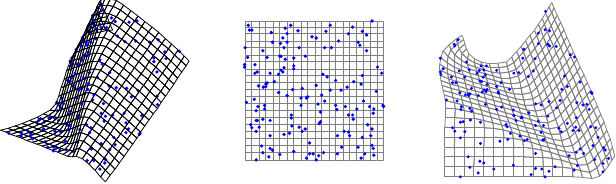Several algorithms are compared in our experiments:

• SOCPimg: our point-wise method described in section 7.3.2;
• FFDref: our smooth reconstruction algorithm described in section 7.4.2;
• FFDinit: the initial solution of our smooth reconstruction algorithm, as described in section 7.4.2;
• Salz: the convex formulation proposed in (166). This method is similar to SOCPimg except for the noise that is not handled the same way. In (166), the author minimizes a cost function that includes a reprojection error' in order to cope with the noise. In SOCPimg, the noise is handled with hard constraints.
• PerrioInit: the upper depth bound' approach of (143,144) which is a point-wise algorithm that iteratively enforces the inextensibility constraints ;
• PerrioRef: the refined approach' of (143,144) which minimizes a cost function resulting in a refined estimation of the 3D points obtained with PerrioInit.

### Reconstruction Errors

The discrepancy between the reconstructed and the ground truth surfaces are quantified with two measures, depending on the surface model used by the algorithms. The point-wise reconstruction error (PWRE), denoted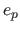, can be used for all the algorithms. It is defined by: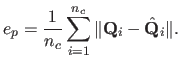(7.16)

For algorithms that uses more complex surface models, such as triangular meshes or FFD, we measures the surface reconstruction error (SRE), denoted. It is the difference between the reconstructed surfaceand the ground truth surface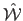:(7.17)

In this experiment, we use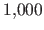randomly generated paper sheets with 150 points correspondences. Figure 7.3 (a) shows the PWRE for all the algorithms and figure 7.3 (b) shows the SRE for the algorithms that use a complex surface model. The main result of this experiment is that our approach FFDref gives the smallest reconstruction errors (PWRE and SRE). Globally, the methods that use complex surface models get better results than the point-wise approaches.

Figure 7.3: Comparison of the reconstruction errors for different algorithms. The central red line is the median. The limits of the blue box are the 25th and the 75th percentiles. The black whiskers' cover approximately 99.3% of the experiment outcomes. The green crosses are the maximal errors over the 1000 trials.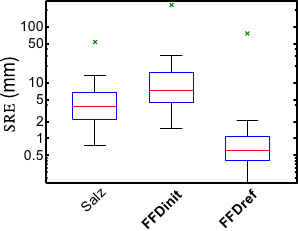(a) Point-wise reconstruction error (b) Surface reconstruction error

### Length of Geodesics

When a reconstructed 3D surface is reconstructed in a truly inextensible way, the transformation of the straight line linking two points in the template image must be the geodesic linking the corresponding two 3D points on the surface. In particular, the length of these two paths must be identical. Testing this hypothesis for our algorithms FFDinit and FFDref is the goal of this experiment. To do so, we use the same data than in the previous experiment. For each surface, we choose randomlypairs of points in the template image. For each pair of points, the length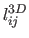of the deformed path linking the 3D pointsand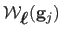on the surface is approximated with the following formula: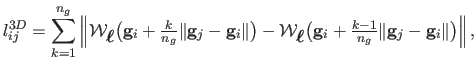(7.18)

where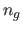is the number of intermediate points used for the approximation (we use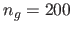since we experimentally observed that the approximation stabilizes for values ofgreater than 180). The lengths of the deformed paths are plotted against their reference length in the template image in figure 7.4 (a) for FFDinit and in figure 7.4 (b,c) for FFDref. Figure 7.4 (b) and figure 7.4 (c) show that, with the surfaces reconstructed with FFDref, the length of the deformed paths are almost equal to the length they should have if they were actual geodesics. In other words, our approach FFDref reconstructs 3D surfaces which are truly inextensible. On the other hand, figure 7.4 (a) shows that the initial solution FFDinit (which is just an FFD fitted to a sparse set of reconstructed 3D points) seems to be much less inextensible.
Figure 7.4: Plot of the length of deformed paths against the length they should have if the reconstructed surface was truly inextensible. The red diagonal line is the place where all the blue points should be for inextensible surfaces.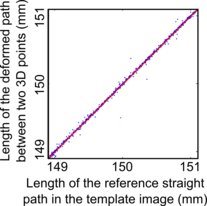(a) FFDinit (b) FFDref (c) Magnification of (b)

Letbe the Euclidean distance between the points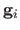and. Table 7.2 gives some statistics on the relative error between the computed lengthand the reference length, i.e. the quantity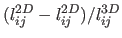. These numbers confirm the results seen in figure 7.4.

Table 7.2: Statistics on the relative errors between the length of transformed paths and the length they should have.
 FFDinit FFDref Mean Std deviation Median Minimun Maximum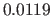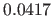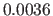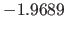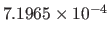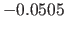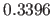### Gaussian curvature

The Gaussian curvature is the product of the two principal curvature (which are the reciprocal of the radius of the osculating circle). For an inextensible surface, the Gaussian curvature is null. In this experiment, we check if this property is satisfied by the smooth surfaces reconstructed with FFDinit and FFDref. We used the samereconstructed surfaces than in the previous experiment. The Gaussian curvature, denoted, is computed forrandomly chosen points on the surface with the formula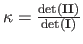, whereand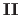are the first and the second fundamental forms of the parametric surface (86). The results of this experiment are reported in table 7.3. It shows that, in average, the Gaussian curvature of the surfaces reconstructed using FFDref are consistently close to 0. It also shows that FFDref gives Gaussian curvatures which are 100 times smaller than the ones obtained with FFDinit. These results demonstrate that the surfaces reconstructed with our approach FFDref are indeed inextensible. Note that this kind of experiment cannot be achieved if a smooth surface is not available.

Table 7.3: Statistics on the (absolute value of the) Gaussian curvatures forreconstructed surfaces andpoints per surface.
 FFDinit FFDref Mean Std deviation Median Minimun Maximum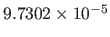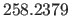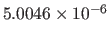## Experiments on Real Data

The algorithms used in the synthetic experiments of section 7.5.1 are applied to real data in figure 7.5 and figure 7.6. These figures shows that our approaches gives good results on real data. In particular, figure 7.5 shows that our method FFDref outperforms the other approaches in the presence of a self-occlusion. This comes from the fact that FFDref requires the surface to be inextensible everywhere, even if there are no point correspondences (which is the case on the self-occluded part of the paper sheet). An accurate stereo reconstruction of the surface in figure 7.6 were available. We compare in table 7.4 the average 3D errors between the surfaces reconstructed with a monocular approach to the stereo reconstruction. Again, our method FFDref is the one giving the best results.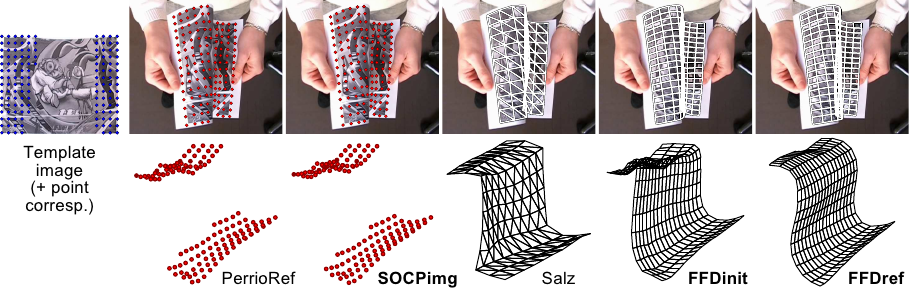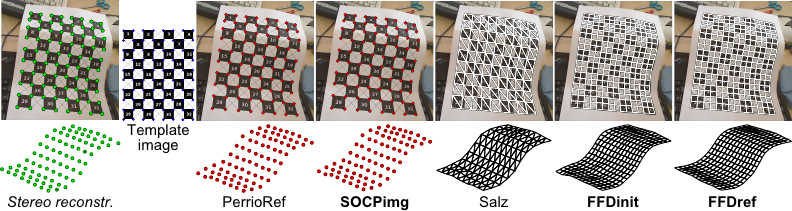Table 7.4: Average 3D error (in millimeters) with respect to the stereo reconstruction of the surface for the surfaces of figure 7.6.
PerrioRef SOCPimg Salz FFDinit FFDref
2.388 2.261 4.743 2.259 1.991

Contributions to Parametric Image Registration and 3D Surface Reconstruction (Ph.D. dissertation, November 2010) - Florent Brunet
Webpage generated on July 2011